# Shapes¶

`rect`(x, y, width, height, roundness=0, draw=True)

Draw a rectangle.```fill(0.95, 0.75, 0)
rect(10, 10, 35, 35)
# see how roundness affects the shape
rect(55, 10, 35, 35, 0.3)
rect(10, 55, 35, 35, 0.7)
rect(55, 55, 35, 35, 1)
```
`rectmode`(mode=None)

Change the way rectangles are specified. Each mode alters the parameters necessary to draw a rectangle using the `rect()` function.

There are 3 different modes available, each expecting different parameters:

• CORNER mode (default) – origin point and dimensions (width and height)

• x-value of the top left corner

• y-value of the top left corner

• width

• height

• CENTER mode – draw a shape centered on a point

• x-coordinate of the rectangle’s center point

• y-coordinate of the rectangle’s center point

• width

• height

• CORNERS mode – origin point and destination point

• x-coordinate of the top left corner

• y-coordinate of the top left corner

• x-coordinate of the bottom right corner

• y-coordinate of the bottom right corner

So while you always specify 4 parameters to the `rect()` function, you can use `rectmode()` to change the function’s behaviour according to what might suit your script’s needs.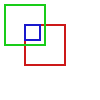```nofill()
strokewidth(2)

rectmode(CORNER)  # default, red
stroke(0.8, 0.1, 0.1)
rect(25, 25, 40, 40)

rectmode(CENTER)  # green
stroke(0.1, 0.8, 0.1)
rect(25, 25, 40, 40)

rectmode(CORNERS)  # blue
stroke(0.1, 0.1, 0.8)
rect(25, 25, 40, 40)
```
`ellipse`(x, y, width, height, draw=True)

Draw an ellipse by specifying the coordinates of its top left origin point, along with its width and height dimensions. See `ellipsemode()` for other ways of drawing ellipses.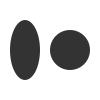```ellipse(10, 20, 30, 60)
ellipse(50, 30, 40, 40) # circle
```

This command is identical to the `oval()` command from Nodebox, which is also supported and works the same.

`ellipsemode`(mode=None)

Change the way ellipses are specified. Each mode alters the parameters necessary to draw an ellipse using the `ellipse()` function.

It works exactly the same as the `rectmode()` command.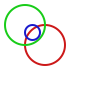```nofill()
strokewidth(2)

ellipsemode(CORNER)  # default, red
stroke(0.8, 0.1, 0.1)
ellipse(25, 25, 40, 40)

ellipsemode(CENTER)  # green
stroke(0.1, 0.8, 0.1)
ellipse(25, 25, 40, 40)

ellipsemode(CORNERS)  # blue
stroke(0.1, 0.1, 0.8)
ellipse(25, 25, 40, 40)
```
`line`(x1, y1, x2, y2, draw=True)

Draw a line from (x1,y1) to (x2,y2).```stroke(0.5)
strokewidth(5)
line(20, 20, 80, 80)
line(20, 80, 80, 20)
line(50, 20, 50, 80)
```
`arc`(x, y, radius, angle1, angle2, type=CHORD, draw=True)

Draws a circular arc with center at (x,y) between two angles.

The default arc type (CHORD) only draws the contour of the circle arc section. The PIE arc type will close the path connecting the arc points to its center, as a pie-chart-like shape.```nofill()
stroke(.2)
autoclosepath(False)
arc(50, 50, 40, 0, 180)
arc(50, 50, 30, -90, 0)
stroke('#ff6633')
arc(50, 50, 20, 0, 270, type=PIE)
```
`arrow`(x, y, width, type=NORMAL, draw=True)

Draw an arrow with its tip at (x,y) and the specified width. Its type can be NORMAL (default) or FORTYFIVE.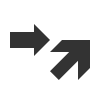```arrow(50, 40, 40)
arrow(90, 40, 40, FORTYFIVE)
```
`star`(x, y, points=20, outer=100, inner=50, draw=True)

Draw a star-like polygon with its center at (x,y).

Following the coordinates, this command expects the number of points, the outer radius of the star shape, and finally the inner radius.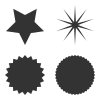```star(25, 25, 5, 20, 10)  # top left
star(75, 25, 10, 20, 3)  # top right
star(25, 75, 20, 20, 17) # bottom left
star(75, 75, 40, 20, 19) # bottom right
```